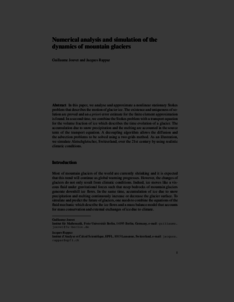Repository: Freie Universität Berlin, Math Department

# Numerical analysis and simulation of the dynamics of mountain glaciers

Jouvet, G. and Rappaz, J. (2014) Numerical analysis and simulation of the dynamics of mountain glaciers. In: Modeling, Simulation and Optimization for Science and Technology. Computational Methods in Applied Sciences (34). Springer Netherlands, pp. 83-92. ISBN 978-94-017-9053-6Preview

522kB

Official URL: https://dx.doi.org/10.1007/978-94-017-9054-3_5

## Abstract

In this chapter, we analyze and approximate a nonlinear stationary Stokes problem that describes the motion of glacier ice. The existence and uniqueness of solutions are proved and an a priori error estimate for the finite element approximation is found. In a second time, we combine the Stokes problem with a transport equation for the volume fraction of ice, which describes the time evolution of a glacier. The accumulation due to snow precipitation and melting are accounted for in the source term of the transport equation. A decoupling algorithm allows the diffusion and the advection problems to be solved using a two-grids method. As an illustration, we simulate the evolution of Aletsch glacier, Switzerland, over the 21st century by using realistic climatic conditions.

Item Type: Book Section Mathematical and Computer Sciences > Mathematics > Numerical Analysis Department of Mathematics and Computer Science > Institute of Mathematics 1863 Ekaterina Engel 13 Apr 2016 08:34 03 Mar 2017 14:42

Repository Staff Only: item control page